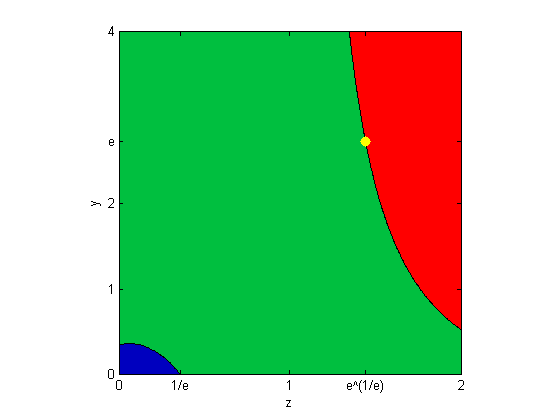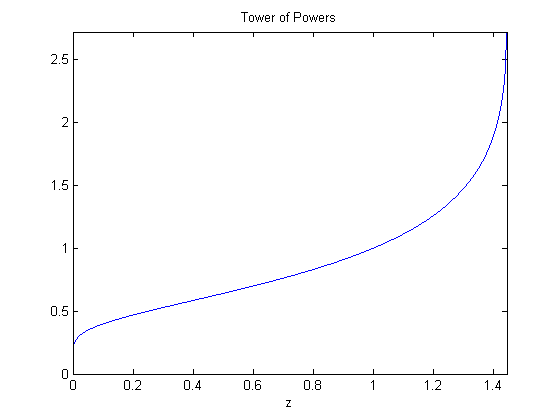Tower of Powers

I first investigated the tower of powers

$$z^{z^{z^z}}$$

when I was in junior high school and was about 14 or 15 years old. I return to it every few decades. This is another one of those episodes.

Contents

Associativity

Does

$$2^{3^4}$$

mean

$${(2^3)}^4 = 8^4 = 2^{12}, \ \mbox{or}, \ 2^{(3^4)} = 2^{81}$$

It makes a big difference.

Addition and multiplication are associative. By that we mean

2*3*4
ans =
24

could have been computed from either

(2*3)*4
ans =
24

or

2*(3*4)
ans =
24

They're both the same.

But subtraction, division, and exponentiation are not associative. MATLAB associates from left to right. So

format rat
2/3/4
ans =
1/6

was computed by

(2/3)/4
ans =
1/6

and not by

2/(3/4)
ans =
8/3

Mathematical convention

The usual mathematical convention for exponentiation is right associativity.

$$a^{b^c} = a^{(b^c)}$$

This is because left associativity can be replaced by multiplication.

$${(a^b)}^c = a^{bc}$$

So, my tower of powers is

$$z^{z^{z^z}} = z^{(z^{(z^z)})}$$

And, I want to make this tower infinitely high.

WS Algorithm

I've been stretching my typographical luck with superscripts and parentheses here. Let's settle down.

For a given value of $z$, let $y$ denote the infinite extension of the tower at the end of the last paragraph. Then, since it's infinite, and associated to the right, we have

$$y = z^y$$

Now that's at least easier to typeset.

There is an obvious way to try to compute it. I call this the WS or World's Simplest algorithm.

$$y_1 = 1$$

$$\mbox{for} \ n = 1, ..., \ \ y_{n+1} = z^{y_n}$$

Of course, this is better known as the Fixed Point algorithm

$$y_{n+1} = f(y_n)$$

for the function

$$f(z) = z^y$$.

Two experiments

Let's try two different values of $z$, $1.25$ and $1.50$.

format long
z = 1.25
y = 1
for n = 1:30
y = z^y;
disp(y)
end
snapnow
z =
1.250000000000000
y =
1
1.250000000000000
1.321714079300705
1.343034993578008
1.349439873733169
1.351369882459350
1.351952000918108
1.352127625454628
1.352180615675240
1.352196604529415
1.352201428918186
1.352202884606055
1.352203323838621
1.352203456370664
1.352203496360284
1.352203508426572
1.352203512067399
1.352203513165966
1.352203513497443
1.352203513597461
1.352203513627640
1.352203513636746
1.352203513639493
1.352203513640323
1.352203513640573
1.352203513640648
1.352203513640671
1.352203513640678
1.352203513640680
1.352203513640681
1.352203513640681
format long
z = 1.5
y = 1
for n = 1:15
y = z^y;
disp(y)
end
snapnow
z =
1.500000000000000
y =
1
1.500000000000000
1.837117307087384
2.106203352148880
2.349005318611934
2.592025704907509
2.860441497460648
3.189324761899238
3.644283987904574
4.382546732246116
5.911914873330858
10.990982932689437
86.181891743310985
1.499263005586202e+15
Inf
Inf

It converges for $z = 1.25$, but not for $z = 1.5$. Something important is happening between $1.25$ and $1.5$.

sqrt(2)

Let's try $z = \sqrt{2}$

format long
z = sqrt(2)
y = 1
for n = 1:30
y = z^y;
disp(y)
end
z =
1.414213562373095
y =
1
1.414213562373095
1.632526919438153
1.760839555880029
1.840910869291011
1.892712696828511
1.926999701847101
1.950034773805818
1.965664886517319
1.976341754409703
1.983668399303822
1.988711773413954
1.992190882947058
1.994594450712102
1.996256666265859
1.997407001141337
1.998203477508703
1.998755133084593
1.999137310119392
1.999402118324998
1.999585622935682
1.999712796329641
1.999800935492971
1.999862023757784
1.999904364443337
1.999933711582101
1.999954052897822
1.999968152149245
1.999977924873871
1.999984698747096
1.999989394007813

It's converging to $y = 2$, because that's a fixed point.

$$(\sqrt{2})^2 = 2$$

So the crucial point is between $1.4$ and $1.5$.

Highest tower

I remember being fascinating when I first learned that the largest possible value of $z$ is a value just a little larger than $\sqrt{2}$. It is the surprising quantity

$$\bar{\mbox{e}} = \mbox{e}^{1/\mbox{e}}$$

ebar = exp(1)^exp(-1)
ebar =
1.444667861009766

This works because $\bar{\mbox{e}}$ is another fixed point.

$$\bar{\mbox{e}}^{\mbox{e}}=(\mbox{e}^{1/{\mbox{e}}})^{\mbox{e}}=\mbox{e}$$

Let's see why $\bar{\mbox{e}}$ is the largest possible value of $z$.

Analysis

Analysis of the WS algorithm is provided by the mean value theorem.

$$f(y_n) = f(y_{n-1}) + f'(\xi_n)(y_n-y_{n-1})$$

for some $\xi_n$ between $y_n$ and $y_{n-1}$.

In our case, $f'(z) = \ln{z}\ z^y$, so

$$y_{n+1} = y_n + (\ln{z})(z^{\xi_n})(y_n-y_{n-1})$$

This tells us that

$$|y_{n+1}-y_n| \le C |y_n-y_{n-1}|$$

for the quantity

$$C = \mbox{max} |\ln{z}||z^y|$$

where the maximum is taken over the $y$ s and $z$ s in a region including the iterates. If $C$ is less than 1, the iterates get closer together, and so the iteration is a contraction that converges.

Let's do a numerical search by making a contour plot of $(\ln{z})(z^y)$. Use green for the region where $C$ is less than $1$, and red and blue for the regions where it is larger than 1.

[Z,Y] = ndgrid(.01:.01:2.0,.01:.01:4.0);
R = log(Z).*Z.^Y;
contourf(Z,Y,R,[-5:4:-1 1:20:21]);
colormap([0 0 .75;0 .75 .25;1 0 0]);
e = exp(1);
line([ebar ebar],[e e],'marker','.','markersize',24,'color','yellow')
set(gca,'xtick',[.01 1/e 1 e^(1/e) 2])
set(gca,'xticklabel',{'0','1/e','1','e^(1/e)','2'})
set(gca,'ytick',[.01 1 2 e 4])
set(gca,'yticklabel',{'0','1','2','e','4'})
xlabel('z'), ylabel('y')
axis squareStable Towers

If we stay in the green region, where $C$ is less than 1, the WS iteration converges, and the tower is stable. That includes all values of $z$ in the interval

$$1/\mbox{e} \le z \le \mbox{e}^{1/\mbox{e}}$$

Towers Topple

The crucial value that I encountered in junior high is the yellow dot, $z=\bar{\mbox{e}}=\mbox{e}^{1/\mbox{e}}$ with $y=\mbox{e}$. As we approach the dot from the stable region, $f'(z)$ approaches $f'(\bar{\mbox{e}})=1$ and the WS algorithm converges incredibly slowly.

To the right of $z=\bar{\mbox{e}}$, the iteration encounters values of $y$ in the red danger region where C is larger than 1, so it diverges rapidly and the tower collapses quickly, as we saw at $z=1.5$.

Small z

Values of $z$ less than $1/\mbox{e}$, which is about $0.37$, are a little more delicate. We might be in the blue region, or might not. It turns out that values of $z$ greater than $\mbox{e}^{\mbox{-e}}$, which is about $0.066$, still encounter values of $y$ larger enough to keep the iteration in the safe green region, so it converges.

But now try $z = 0.05$ for a few iterations.

z = 0.05
y = 1
for k = 1:20, y = z^y; disp(y), end
z =
0.050000000000000
y =
1
0.050000000000000
0.860891659331735
0.075849745545982
0.796741072475616
0.091921259400909
0.759290007184149
0.102834993569945
0.734866735033395
0.110641061786732
0.717881331845427
0.116416584607138
0.705567440560545
0.120791283462031
0.696381005849372
0.124161635028397
0.689385252311344
0.126791198828070
0.683975974940914
0.128862555680671
0.679744887323325

It doesn't look like it wants to converge. Run 300 more iterations without any output.

for k = 1:300, y = z^y; end

Now check on how we're doing after a total of 320 iterations.

for k = 1:10, y = z^y; disp(y), end
0.137359395737956
0.662660838900549
0.137359395737956
0.662660838900549
0.137359395737956
0.662660838900549
0.137359395737956
0.662660838900549
0.137359395737956
0.662660838900549

It has settled into a pattern, oscillating back and forth between two values. This is a simple example of a period doubling bifurcation.

This is the behavior for all $z$ less than $\mbox{e}^{\mbox{-e}}$. The values of $y$ encountered put us in the blue region. The fixed point iteration is not a contraction. It settles into a stable two-cycle. The distance between the two limit points increases as $z$ gets smaller.

A tower based on a value of $z$ less than $\mbox{e}^{\mbox{-e}}$ will have its odd numbered floors one height and its even numbered floors another.

Enter Lambert W

Here is another way to look at the tower of powers. It involves the Lambert W function from my previous blog.

$$y = z^y = \mbox{e}^{y\ln{z}}$$

$$y \mbox{e}^{-y\ln{z}} = 1$$

$$-y \ln{z} \ \mbox{e}^{-y\ln{z}} = -\ln{z}$$

$$-y \ln{z} = W(-\ln{z})$$

$$y = \frac{W(-\ln{z})}{-\ln{z}}$$

So, the Lambert W function provides a value of the infinite tower for any value of $z$. I didn't know this when I was in junior high school.

The Tower function

Let's try this formulation for a few values of $z$, including some smaller than $\mbox{e}^{\mbox{-e}}$.

T = @(z) Lambert_W(-log(z))./(-log(z))
z = [1.25 sqrt(2) ebar 1.5 e^(-e) 0.05 10.^(-(3:3:15))]';
disp('         z                    T(z)')
disp([z T(z)])
T =
@(z)Lambert_W(-log(z))./(-log(z))
z                    T(z)
1.250000000000000   1.352203513640681
1.414213562373095   2.000000000000000
1.444667861009766   2.718281787975775
1.500000000000000                 NaN
0.065988035845313   0.367879441171442
0.050000000000000   0.350224852743194
0.001000000000000   0.219513151627722
0.000001000000000   0.141526856553182
0.000000001000000   0.107583717152717
0.000000000001000   0.087971500166559
0.000000000000001   0.074997053328098

Plot

And now a plot.

ezplot(T,[0 ebar])
axis([0 ebar 0 e])
title('Tower of Powers')Unstable

The value of $y$ obtained from the Lambert W formulation is a fixed point of $f(z)$, but a possibly unstable one. If we have a value of $z$ less than $\mbox{e}^{\mbox{-e}}$ and we start the fixed point algorithm at the value of $y$ given by the Lambert W formula exactly, it will stay there. But if we start with any other value of $y$, the iterates will eventually find their way to the pair of bifurcation attractors.

Published with MATLAB® R2013b

|